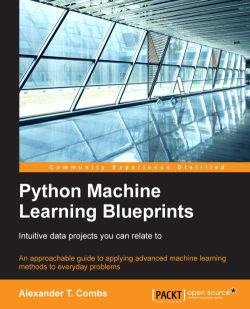### Python Machine Learning Blueprints: Intuitive data projects you can relate to4.5 (11 reviews total)
By Alexander T. Combs
• FREE Subscribe Access now
• £37.99 Print + eBook
• £27.99 eBook
• Constantly updated with 100+ new titles each month
• Breadth and depth in over 1,000+ technologies

Machine Learning is transforming the way we understand and interact with the world around us. But how much do you really understand it? How confident are you interacting with the tools and models that drive it?

Python Machine Learning Blueprints puts your skills and knowledge to the test, guiding you through the development of some awesome machine learning applications and algorithms with real-world examples that demonstrate how to put concepts into practice.

You’ll learn how to use cluster techniques to discover bargain air fares, and apply linear regression to find yourself a cheap apartment – and much more. Everything you learn is backed by a real-world example, whether its data manipulation or statistical modelling.

That way you’re never left floundering in theory – you’ll be simply collecting and analyzing data in a way that makes a real impact.

Publication date:
July 2016
Publisher
Packt
Pages
332
ISBN
9781784394752

## ChapterÂ 1.Â The Python Machine Learning Ecosystem

Machine learning is rapidly changing our world. As the centerpiece of artificial intelligence, it is difficult to go a day without reading how it will transform our lives. Some argue it will lead us into a Singularity-style techno-utopia. Others suggest we are headed towards a techno-pocalypse marked by constant battles with job-stealing robots and drone death squads. But while the pundits may enjoy discussing these hyperbolic futures, the more mundane reality is that machine learning is rapidly becoming a fixture of our daily lives. Through subtle but progressive improvements in how we interact with computers and the world around us, machine learning is quietly improving our lives.

If you shop at online retailers such as Amazon.com, use streaming music or movie services such as Spotify or Netflix, or even just perform a Google search, you have encountered a machine learning application. The data generated by the users of these services is collected, aggregated, and fed into models that improve the services by creating tailored experiences for each user.

Now is an ideal time to dive into developing machine learning applications, and as you will discover, Python is an ideal choice with which to develop these applications. Python has a deep and active developer community, and many of these developers come from the scientific community as well. This has provided Python with a rich array of libraries for scientific computing. In this book, we will discuss and use a number of these libraries from this Python scientific stack.

In the chapters that follow, we'll learn step by step how to build a wide variety of machine learning applications. But before we begin in earnest, we'll spend the remainder of this chapter discussing the features of these key libraries and how to prepare your environment to best utilize them.

We'll cover the following topics in this chapter:

• The data science/machine learning workflow

• Libraries for each stage of the workflow

## The data science/machine learning workflow

Building machine learning applications, while similar in many respects to the standard engineering paradigm, differs in one crucial way: the need to work with data as a raw material. The success of a data project will, in large part, depend on the quality of the data that you acquired as well as how it's handled. And because working with data falls into the domain of data science, it is helpful to understand the data science workflow: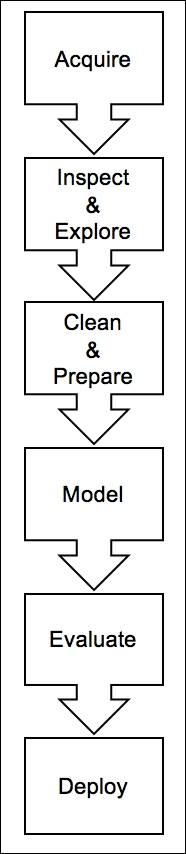The process proceeds through these six steps in the following order: acquisition, inspection and exploration, cleaning and preparation, modeling, evaluation, and finally deployment. There is often the need to circle back to prior steps, such as when inspecting and preparing the data or when evaluating and modeling, but the process at a high level can be described as shown in the preceding diagram.

Let's now discuss each step in detail.

### Acquisition

Data for machine learning applications can come from any number of sources; it may be e-mailed as a CSV file, it may come from pulling down server logs, or it may require building a custom web scraper. The data may also come in any number of formats. In most cases, it will beÂ text-based data, but as we'll see, machine learning applications may just as easily be built utilizing images or even video files. Regardless of the format, once the data is secured,Â it is crucial to understand what's in the dataâ€”as well as what isn't.

### Inspection and exploration

Once the data has been acquired, the next step is to inspect and explore it. At this stage, the primary goal is to sanity-check the data, and the best way to accomplish this is to look for things that are either impossible or highly unlikely. As an example, if the data has a unique identifier, check to see that there is indeed only one; if the data is price-based, check whether it is always positive; and whatever the data type, check the most extreme cases. Do they make sense? A good practice is to run some simple statistical tests on the data and visualize it. Additionally, it is likely that some data is missing or incomplete. It is critical to take note of this during this stage as it will need to be addressed it later during the cleaning and preparation stage. Models are only as good as the data that goes into them, so it is crucial to get this step right.

### Cleaning and preparation

When all the data is in order, the next step is to place it in a format that is amenable to modeling. This stage encompasses a number of processes such as filtering, aggregating, imputing, and transforming. The type of actions that are necessary will be highly dependent on the type of data as well as the type of library and algorithm utilized. For example, with natural-language-based text, the transformations required will be very different from those required for time series data. We'll see a number of examples of these types of transformations throughout the book.

### Modeling

Once the data preparation is complete, the next phase is modeling. In this phase, an appropriate algorithm is selected and a model is trained on the data. There are a number of best practices to adhere to during this stage, and we will discuss them in detail, but the basic steps involve splitting the data into training, testing, and validation sets. This splitting up of the data may seem illogicalâ€”especially when more data typically yields better modelsâ€”but as we'll see, doing this allows us to get better feedback on how the model will perform in the real world, and prevents us from the cardinal sin of modeling: overfitting.

### Evaluation

Once the model is built and making predictions, the next step is to understand how well the model does that. This is the question that evaluation seeks to answer. There are a number of ways to measure the performance of a model, and again it is largely dependent on the type of data and the model used, but on the whole, we are seeking to answer the question of how close are the model's predictions toÂ the actual value. There are arrays of confusing-sounding terms such as root mean-square error, Euclidean distance, andÂ F1 score, but in the end, they are all just a measure of distance between the actual value and the estimated prediction.

### Deployment

Once the model's performance is satisfactory, the next step is deployment. This can take a number of forms depending on the use case, but common scenarios include utilization as a feature within another larger application, a bespoke web application, or even just a simple cron job.

## Python libraries and functions

Now that we have an understanding of each step in the data science workflow, we'll take a look at a selection of useful Python libraries and functions within these libraries for each step.

### Acquisition

Because one of the more common ways of accessing data is through a RESTful API, one library that to be aware of is the Python Requests library (http://www.python-requests.org/en/latest/). Dubbed HTTP for humans, it provides a clean and simple way to interact with APIs.

Let's take a look at a sample interaction using Requests to pull down data from GitHub's API. Here we will make a call to the API and request a list of starred repositories for a user:

```import requests
r = requests.get(r"https://api.github.com/users/acombs/starred")
r.json()
```

This will return a JSON document of all the repositories that the user has starred along with their attributes. Here is a snippet of the output for the preceding call: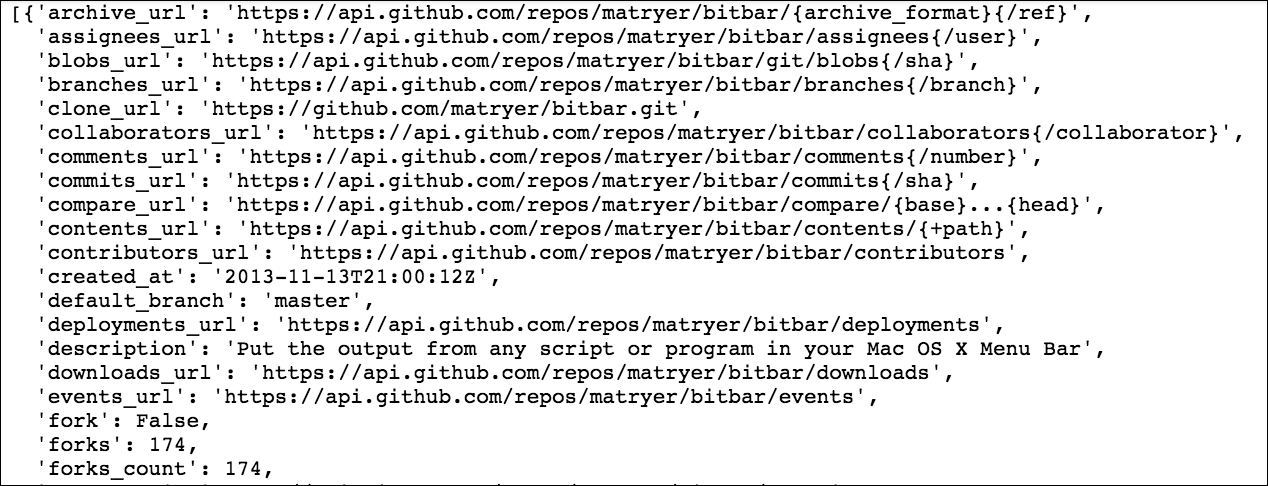The `requests` library has an amazing number of featuresâ€”far too many to cover here, but I do suggest that you check out the documentation in the link provided above.

### Inspection

Because inspecting data is a critical step in the development of machine learning applications, we'll now have an in-depth look at several libraries that will serve us well in this task.

#### The Jupyter notebook

There are a number of libraries that will help ease the data inspection process. The first is the Jupyter notebook with IPython (http://ipython.org/). This is a full-fledged, interactive computing environment that's ideal for data exploration. Unlike most development environments, the Jupyter notebook is a web-based frontend (to the IPython kernel) that is divided into individual code blocks or cells. Cells can be run individually or all at once, depending on the need. This allows the developer to run a scenario, see the output, then step back through the code, make adjustments, and see the resulting changesâ€”all without leaving the notebook. Here is a sample interaction in the Jupyter notebook: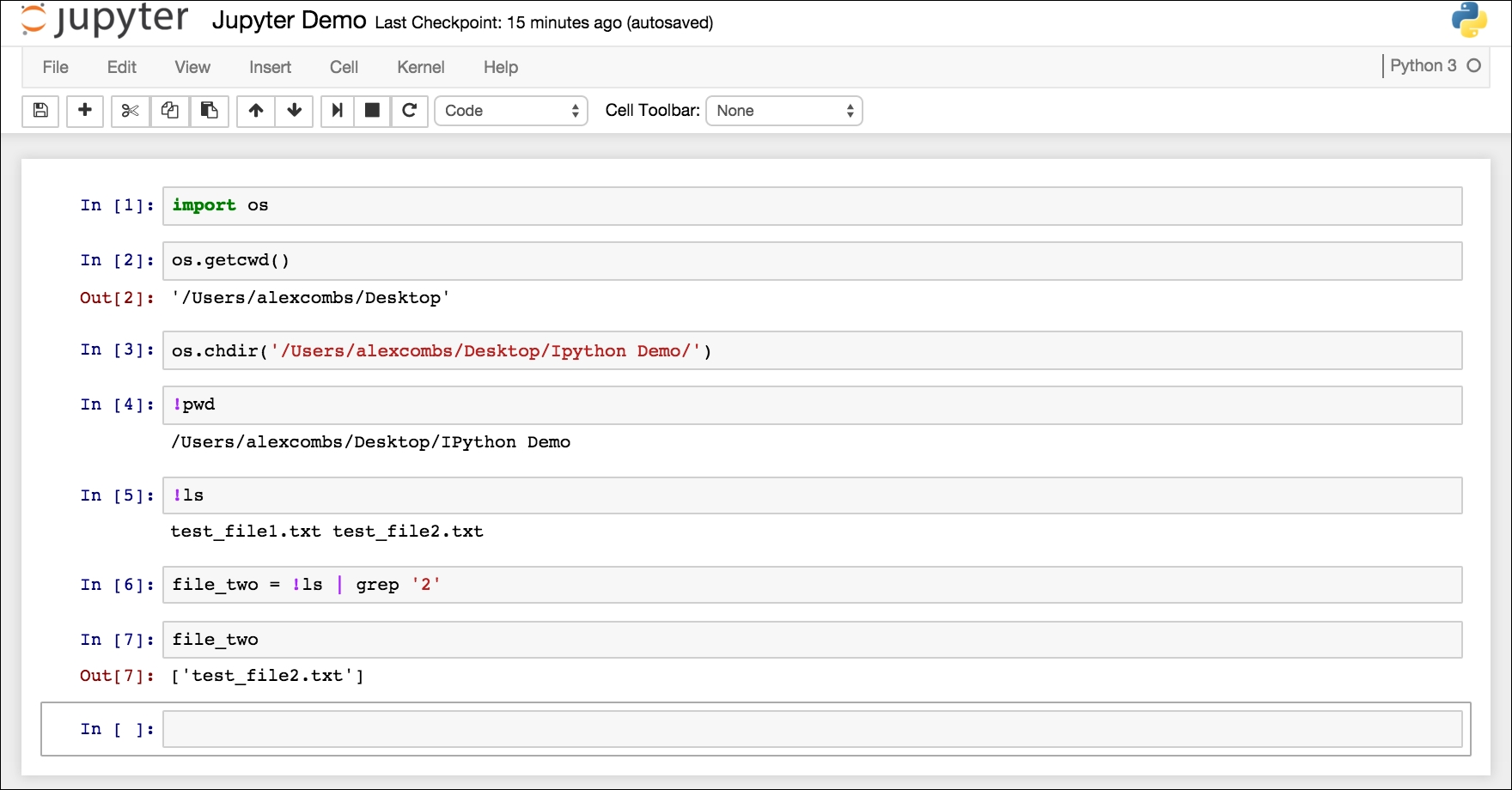Notice that we have done a number of things here and interacted with not only the IPython backend, but the terminal shell as well. This particular instance is running a Python 3.5 kernel, but you can just as easily run a Python 2.X kernel if you prefer. Here, we have imported the Python `os` library and made a call to find the current working directory (cell #2), which you can see is the output below the input code cell. We then changed directories using the `os` library in cell #3, but then stopped utilizing the `os` library and began using Linux-based commands in cell #4. This is done by adding the `!` prepend to the cell. In cell #6, you can see that we were even able to save the shell output to a Python variable (`file_two`). This is a great feature that makes file operations a simple task.

Now let's take a look at some simple data operations using the notebook. This will also be our first introduction to another indispensable library, `pandas`.

#### Pandas

Pandas is a remarkable tool for data analysis. According to the pandas documentation (http://pandas.pydata.org/pandas-docs/version/0.17.1/):

It has the broader goal of becoming the most powerful and flexible open source data analysis/manipulation tool available in any language.

If it doesn't already live up to this claim, it can't be too far off. Let's now take a look:

```import os
import pandas as pd
import requests

PATH = r'/Users/alexcombs/Desktop/iris/'

r = requests.get('https://archive.ics.uci.edu/ml/machine-learning-databases/iris/iris.data')

with open(PATH + 'iris.data', 'w') as f:
f.write(r.text)

os.chdir(PATH)

df = pd.read_csv(PATH + 'iris.data', names=['sepal length', 'sepal width', 'petal length', 'petal width', 'class'])

```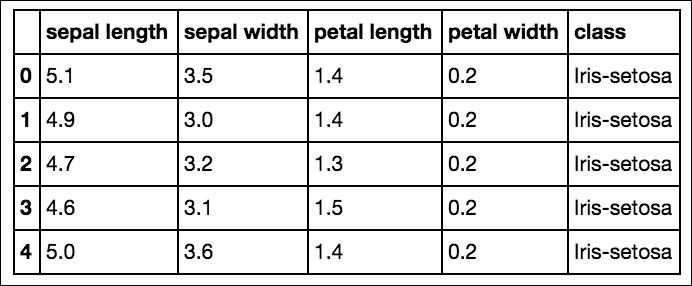As seen in the preceding code and screenshot we have downloaded a classic machine learning dataset, `iris.data`, from https://archive.ics.uci.edu/ml/datasets/Iris and written it into the `iris` directory. This is actually a CSV file, and using Pandas, we made a call to read in the file. We also added column names, as this particular file lacked a header row. If the file did contain a header row, pandas would have automatically parsed and reflected this. Compared to other CSV libraries, pandas makes this a simple operation.

Parsing files is just one small feature of this library. To work with datasets that will fit on a single machine, pandas is the ultimate tool; it is a bit like Excel on steroids. Like the popular spreadsheet program, the basic units of operation are columns and rows of data in the form of tables.Â In the terminology of pandas, columns of data are Series and the table is a DataFrame.

Using the same `iris` DataFrame as shown in the preceding screenshot, let's have a look at a few common operations:

```df['sepal length']
```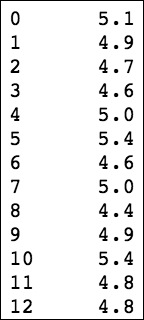The first action was just to select a single column from the DataFrame by referencing it by its column name. Another way that we can perform this data slicing is to use theÂ `.ix[row,column]` notation. Let's select the first two columns and first four rows using this notation:

```df.ix[:3,:2]

```

The preceding code generates the following output: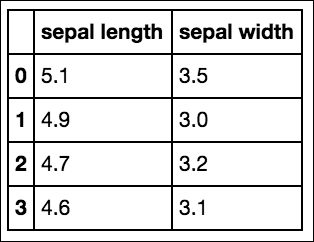Using the `.ix` notation and Python list slicing syntax, we were able to select a slice of this DataFrame. Now let's take it up a notch and use a list iterator to select just the width columns:

```df.ix[:3, [x for x in df.columns if 'width' in x]]

```

The preceding code generates the following output: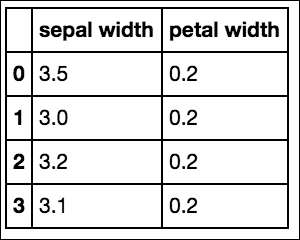What we have done here is create a list that is a subset of all the columns. The precedingÂ `df.columns` returns a list of all the columns and our iteration uses a conditional statement to select only those with `width` in the title. Obviously, in this situation, we could have just as easily typed out the columns that we wanted in a list, but this illustrates the power available when dealing with much larger datasets.

We've seen how to select slices based on their position in the DataFrame, but let's now look at another method to select data. This time we will select a subset of the data based on some specific conditions. We start by listing all the available unique classes and then selecting one of these:

```df['class'].unique()

```

The preceding code generates the following output: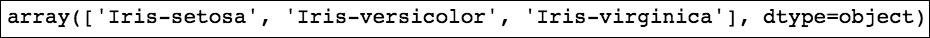```df[df['class']=='Iris-virginica']
```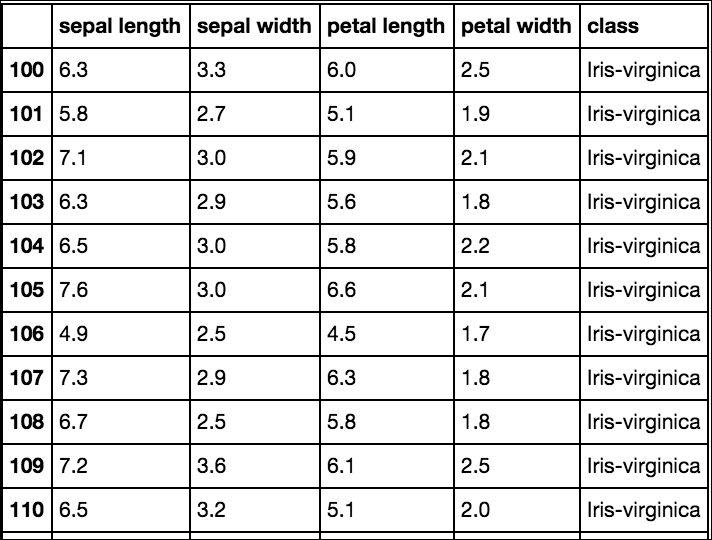In the far right-hand column, we can see that our DataFrame only contains data for the `Iris-virginica` class. In fact, the size of the DataFrame is now 50 rows, down from the original 150 rows:

```df.count()

```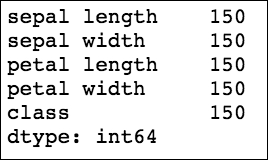```df[df['class']=='Iris-virginica'].count()

```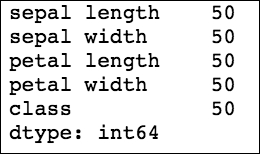WeÂ can also see that the index on the left retains the original row numbers. We can now save this data as a new DataFrame and reset the index, as shown in the following code and screenshot:

```virginica = df[df['class']=='Iris-virginica'].reset_index(drop=True)
virginica

```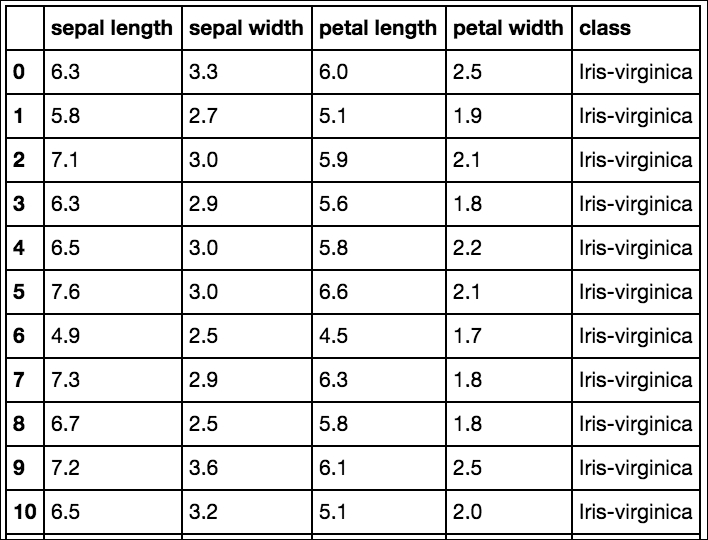We have selected data by placing a condition on one column; let's now add more conditions. We'll go back to our original DataFrame and select data using two conditions:

``` df[(df['class']=='Iris-virginica')&(df['petal width']>2.2)]

```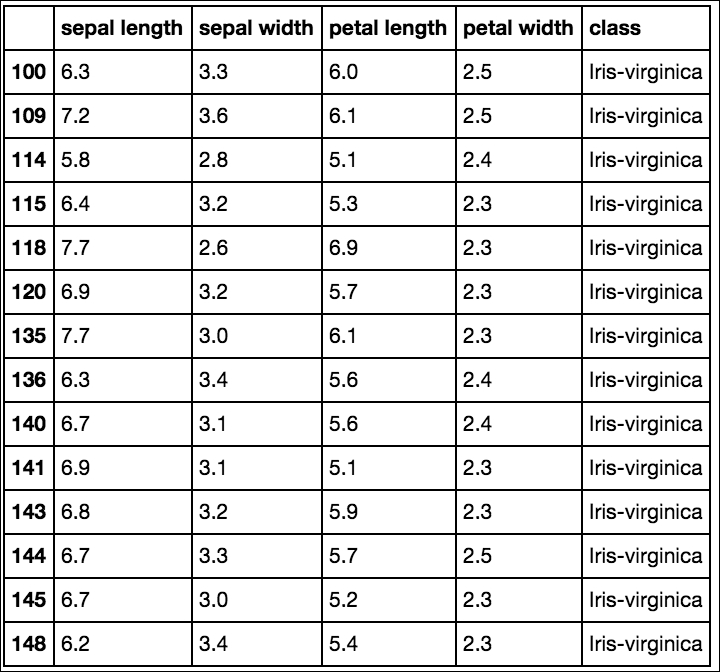The DataFrame now includes data only from the `Iris-virginica` class with a petal width greater than 2.2.

Let's now use pandas to get some quick descriptive statistics from our Iris dataset:

```df.describe()

```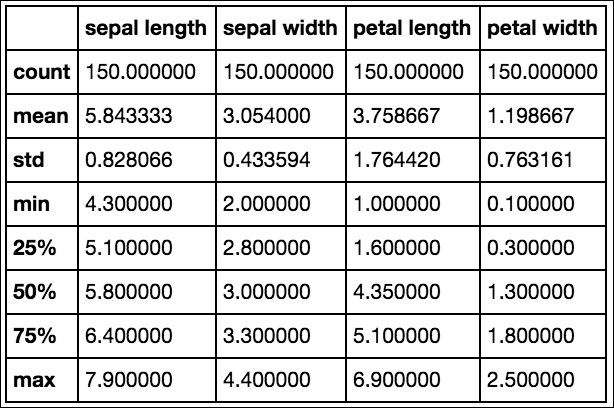With a call to the DataFrameÂ `.describe()` method, we received a breakdown of the descriptive statistics for each of the relevant columns. (Notice that class was automatically removed as it is not relevant for this.)

We could also pass in custom percentiles if we wanted more granular information:

``` df.describe(percentiles=[.20,.40,.80,.90,.95])

```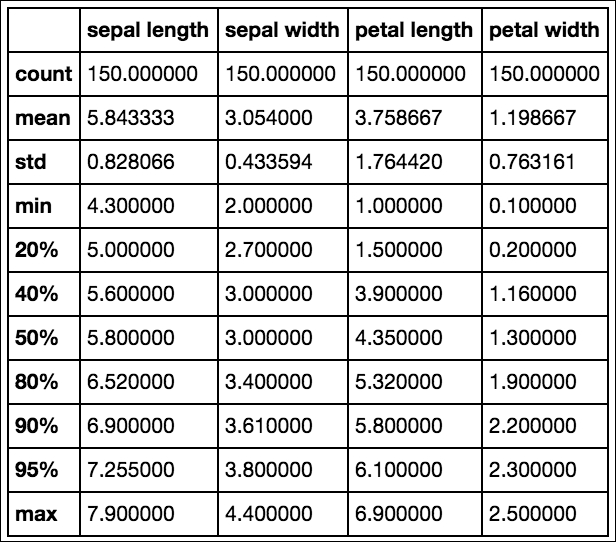Next, let's check whether there is any correlation between these features. This can be done by calling `.corr()` on our DataFrame:

```df.corr()

```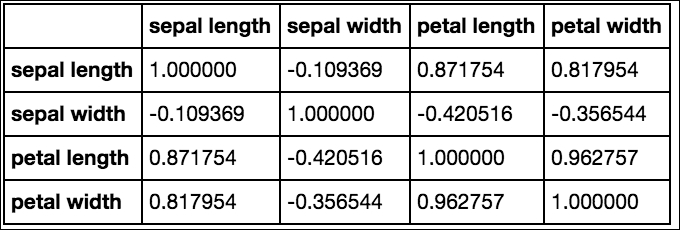The default returns the Pearson correlation coefficient for each row-column pair. This can be switched to Kendall's tau or Spearman's rank correlation coefficient by passing in a method argument (for instance, `.corr(method="spearman")` or `.corr(method="kendall")`).

#### Visualization

So far, we have seen how to select portions of a DataFrame and get summary statistics from our data, but let's now move on to learning how to visually inspect the data. But first, why even bother with visual inspection? Let's see an example to understand why.

The following table illustrates the summary statistics for four distinct series of x and y values:

 Series of x and y Values Mean of x 9 Mean of y 7.5 Sample variance of x 11 Sample variance of y 4.1 Correlation between x and y 0.816 Regression line y=3.00+0.500x

Based on the series having identical summary statistics, we might assume that these series would appear visually similar. We would, of course, be wrong. Very wrong. The four series are part of Anscombe's quartet, and they were deliberately created to illustrate the importance of visual data inspection. Each series is plotted in the following image: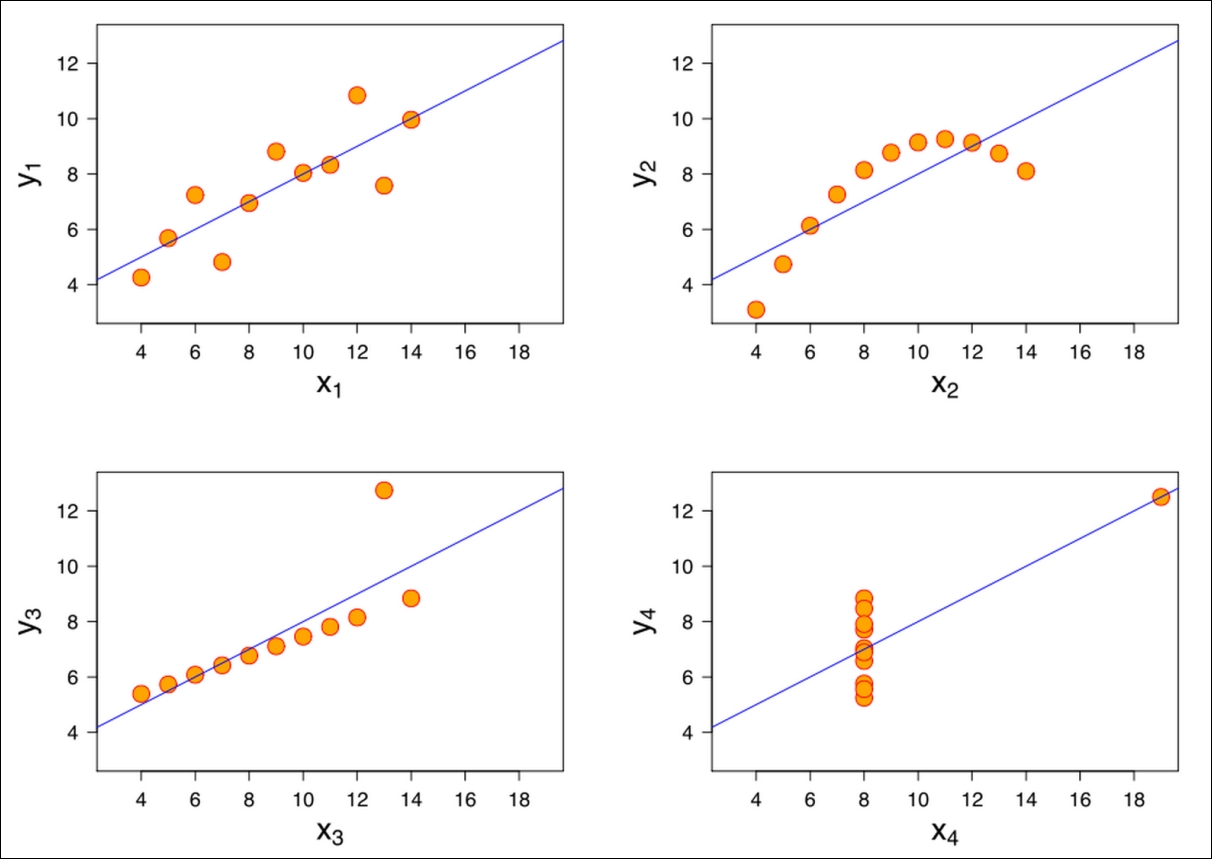The Anscombe's quartet taken from https://en.wikipedia.org/wiki/Anscombe%27s_quartet.

Clearly, we would not treat these datasets as identical after having visualized them. So now that we understand the importance of visualization, let's take a look at a pair of useful Python libraries for this.

##### The matplotlib library

The first library that we'll take a look at is `matplotlib`. It is the great grandfather of Python plotting libraries. Originally created to emulate the plotting functionality of MATLAB, it grew into a fully-featured library in its own right with an enormous range of functionality. For those that have not come from a MATLAB background, it can be hard to understand how all the pieces work together to create the graphs.

We'll break down the pieces into logical components to make sense of what's going on. Before diving into `matplotlib` in full, let's set up our Jupyter notebook to allow us to see our graphs in line. To do this, we'll need to add the following lines to our `import` statements:

```import matplotlib.pyplot as plt
plt.style.use('ggplot')
%matplotlib inline
import numpy as np
```

The first line imports `matplotlib`, the second line sets the styling to approximate R's `ggplot` library (this requires matplotlib 1.41), the third line sets the plots so that they are visible in the notebook, and the final line imports `numpy`. We'll use `numpy` for a number of operations later in the chapter.

Now, let's generate our first graph on the Iris dataset using the following code:

```fig, ax = plt.subplots(figsize=(6,4))
ax.hist(df['petal width'], color='black');
ax.set_ylabel('Count', fontsize=12)
ax.set_xlabel('Width', fontsize=12)
plt.title('Iris Petal Width', fontsize=14, y=1.01)

```

The preceding code generates the following output: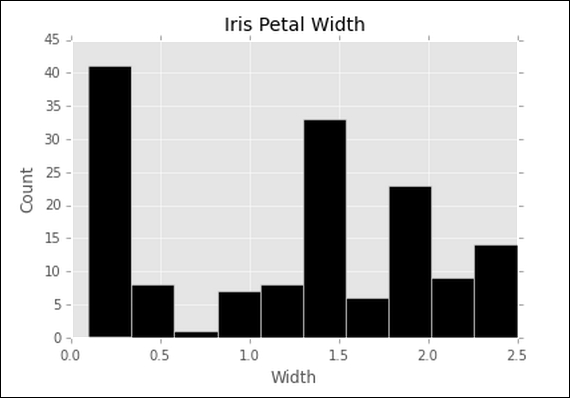There is a lot going on even in this simple example, but we'll break it down line by line. The first line creates a single subplot with a width of 6" and height of 4". We then plot a histogram of the petal width from our `iris` DataFrame by calling `.hist()` and passing in our data. We also set the bar color to `black` here. The next two lines place labels on our y and x axes, respectively, and the final line sets the title for our graph. We tweak the title's y position relative to the top of the graph with the `y` parameter and increase the font size slightly over the default. This gives us a nice histogram of our petal width data. Let's now expand on this and generate histograms for each column of our `iris` dataset:

```fig, ax = plt.subplots(2,2, figsize=(6,4))

ax.hist(df['petal width'], color='black');
ax.set_ylabel('Count', fontsize=12)
ax.set_xlabel('Width', fontsize=12)
ax.set_title('Iris Petal Width', fontsize=14, y=1.01)

ax.hist(df['petal length'], color='black');
ax.set_ylabel('Count', fontsize=12)
ax.set_xlabel('Lenth', fontsize=12)
ax.set_title('Iris Petal Lenth', fontsize=14, y=1.01)

ax.hist(df['sepal width'], color='black');
ax.set_ylabel('Count', fontsize=12)
ax.set_xlabel('Width', fontsize=12)
ax.set_title('Iris Sepal Width', fontsize=14, y=1.01)

ax.hist(df['sepal length'], color='black');
ax.set_ylabel('Count', fontsize=12)
ax.set_xlabel('Length', fontsize=12)
ax.set_title('Iris Sepal Length', fontsize=14, y=1.01)
plt.tight_layout()

```

The output for the preceding code is shown in the following screenshot: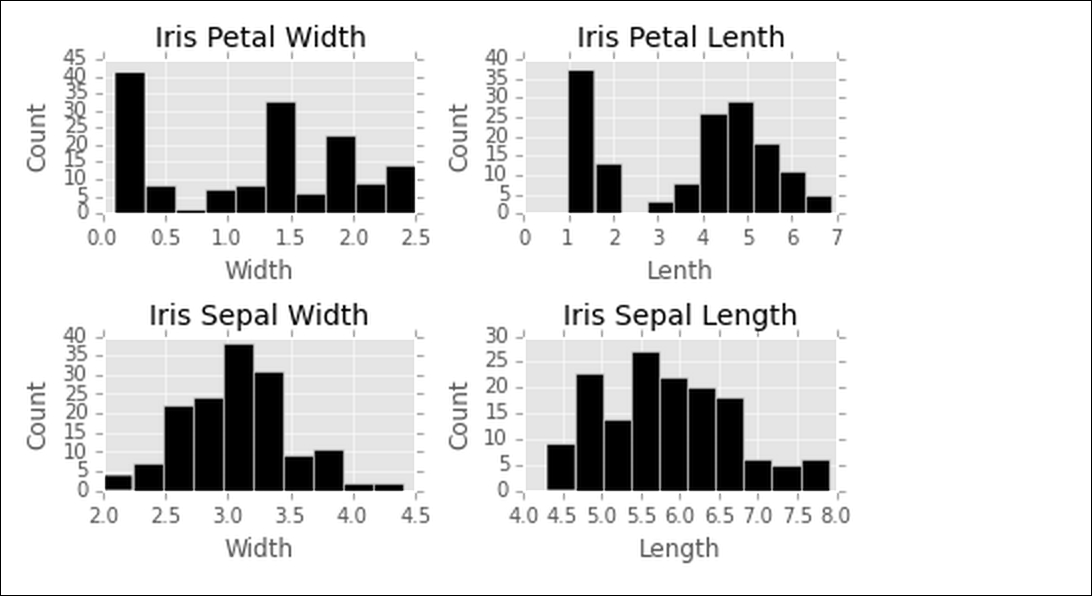Obviously, this is not the most efficient way to code this, but it is useful to demonstrate how `matplotlib` works. Notice that instead of the single subplot object, `ax`, that we had in the first example, we now have four subplots, which are accessed through what is now the `ax` array. A new addition to the code is the call to `plt.tight_layout()`; this method will nicely adjust the subplots automatically to avoid crowding.

Let's now take a look at a few other types of plots available in `matplotlib`. One useful plot is a scatterplot. Here, we will plot the petal width against the petal length:

```fig, ax = plt.subplots(figsize=(6,6))
ax.scatter(df['petal width'],df['petal length'], color='green')
ax.set_xlabel('Petal Width')
ax.set_ylabel('Petal Length')
ax.set_title('Petal Scatterplot')
```

The preceding code generates the following output: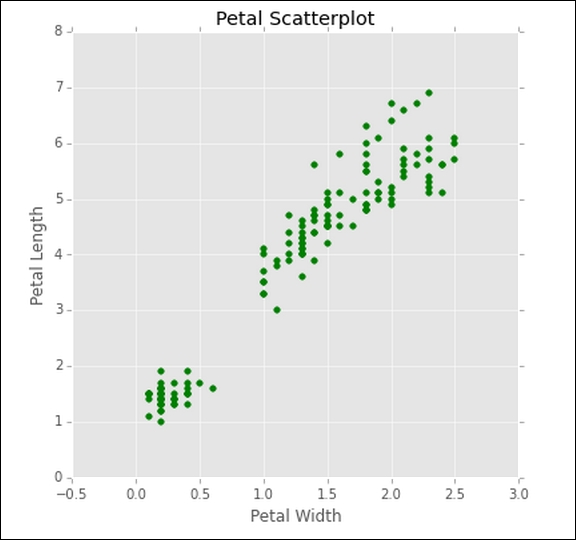As explained earlier, we could add multiple subplots to examine each facet.

Another plot we could examine is a simple line plot. Here we look at a plot of the petal length:

```fig, ax = plt.subplots(figsize=(6,6))
ax.plot(df['petal length'], color='blue')
ax.set_xlabel('Specimen Number')
ax.set_ylabel('Petal Length')
ax.set_title('Petal Length Plot')
```

The preceding code generates the following output: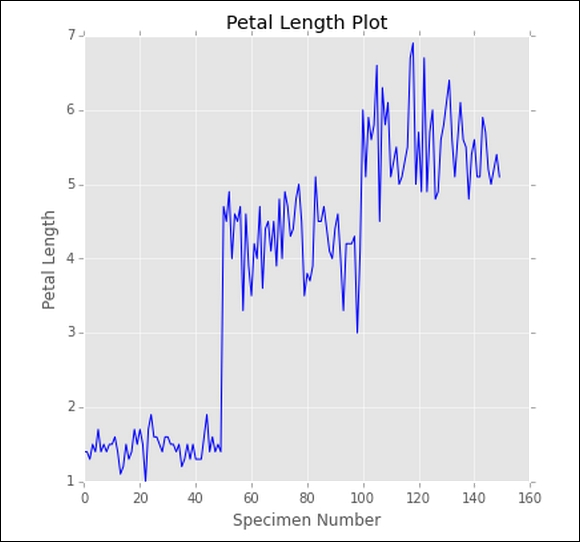Based on this simple line plot, we can already see that there are distinctive clusters of lengths for each classâ€”remember that our sample dataset had 50 ordered examples of each class. This tells us that the petal length is likely to be a useful feature to discriminate between classes.

Let's look at one final type of chart from the `matplotlib` library, the bar chart. This is perhaps one of the more common charts that you'll see. Here, we'll plot a bar chart for the mean of each feature for the three classes of irises, and to make it more interesting, we'll make it a stacked bar chart with a number of additional `matplotlib` features:

```fig, ax = plt.subplots(figsize=(6,6))
bar_width = .8
labels = [x for x in df.columns if 'length' in x or 'width' in x]
ver_y = [df[df['class']=='Iris-versicolor'][x].mean() for x in
labels]
vir_y = [df[df['class']=='Iris-virginica'][x].mean() for x in
labels]
set_y = [df[df['class']=='Iris-setosa'][x].mean() for x in labels]
x = np.arange(len(labels))
ax.bar(x, vir_y, bar_width, bottom=set_y, color='darkgrey')
ax.bar(x, set_y, bar_width, bottom=ver_y, color='white')
ax.bar(x, ver_y, bar_width, color='black')
ax.set_xticks(x + (bar_width/2))
ax.set_xticklabels(labels, rotation=-70, fontsize=12);
ax.set_title('Mean Feature Measurement By Class', y=1.01)
ax.legend(['Virginica','Setosa','Versicolor'])

```

The preceding code generates the following output: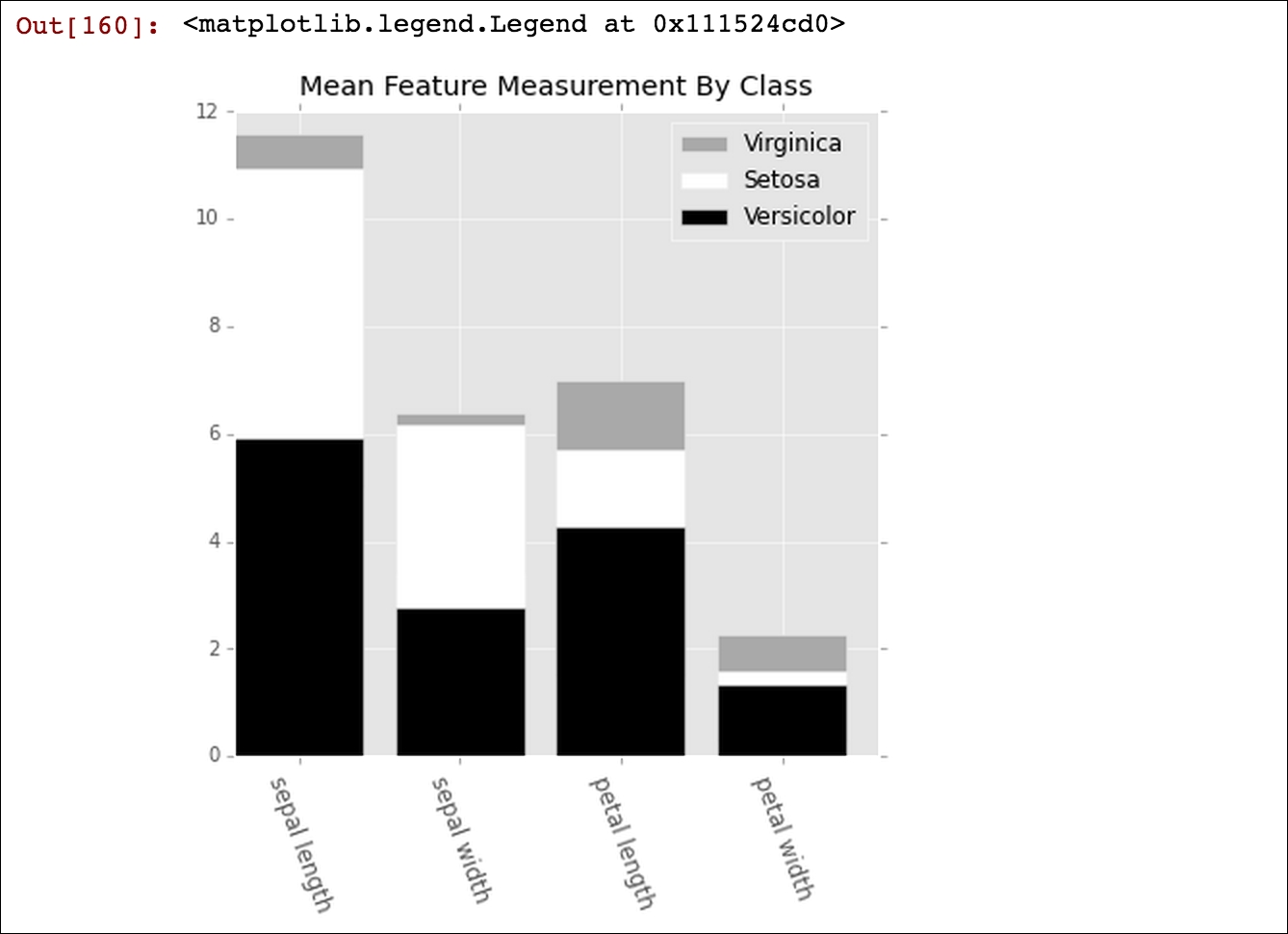To generate the bar chart, we need to pass the `x` and `y` values to the `.bar()` method. In this case, the `x` values will just be an array of the length of the features that we are interested inâ€”four here, or one for each column in our DataFrame. The `np.arange()` function is an easy way to generate this, but we could nearly as easily input this array manually. As we don't want the x axis to display this 1 through 4, we call the `.set_xticklabels()` method and pass in the column names that we want to display. To line up the `x` labels properly, we also need to adjust the spacing of the labels; this is why we set `xticks` to `x` plus half the size of `bar_width`, which we also set earlier at `0.8`. The `y` values come from taking the mean of each feature for each class. We then plot each by calling `.bar()`. It is important to note that we pass in a `bottom` parameter for each series that sets its minimum y point equal to the maximum y point of the series below it. This creates the stacked bars. Finally, we add a legend that describes each series. The names are inserted into the legend list in order of the placement of the bars from top to bottom.

##### The seaborn library

The next visualization library that we'll look at is called `seaborn` (http://stanford.edu/~mwaskom/software/seaborn/index.html). It is a library that was created specifically for statistical visualizations. In fact, it is perfect for use with `pandas` DataFrames where the columns are features and rows are observations. This style of DataFrame is called tidy data, and it is the most common form for machine learning applications.

Let's now take a look at the power of `seaborn`:

```import seaborn as sns
sns.pairplot(df, hue="class")
```

With just these two lines of code, we get the following output: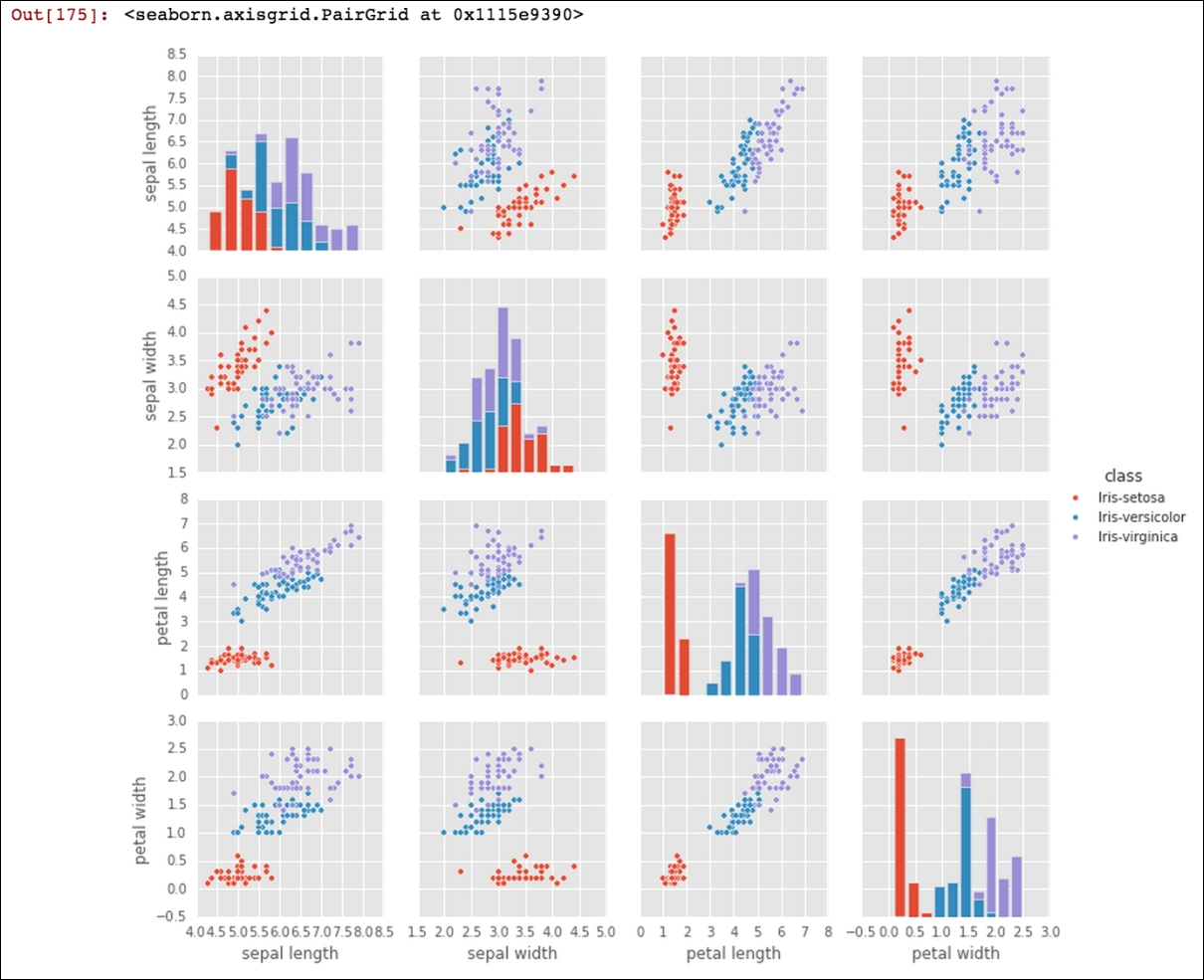Having just detailed the intricate nuances of `matplotlib`, the simplicity with which we generated this plot is notable. All of our features have been plotted against each other and properly labeled with just two lines of code. Was learning pages of `matplotlib` a waste, when `seaborn` makes these types of visualizations so simple? Fortunately, that isn't the case as `seaborn` is built on top of `matplotlib`. In fact, we can use all of what we learned about `matplotlib` to modify and work with `seaborn`. Let's take a look at another visualization:

```fig, ax = plt.subplots(2, 2, figsize=(7, 7))
sns.set(style='white', palette='muted')
sns.violinplot(x=df['class'], y=df['sepal length'], ax=ax[0,0])
sns.violinplot(x=df['class'], y=df['sepal width'], ax=ax[0,1])
sns.violinplot(x=df['class'], y=df['petal length'], ax=ax[1,0])
sns.violinplot(x=df['class'], y=df['petal width'], ax=ax[1,1])
fig.suptitle('Violin Plots', fontsize=16, y=1.03)
for i in ax.flat:
plt.setp(i.get_xticklabels(), rotation=-90)
fig.tight_layout()

```

The preceding lines of code generate the following output: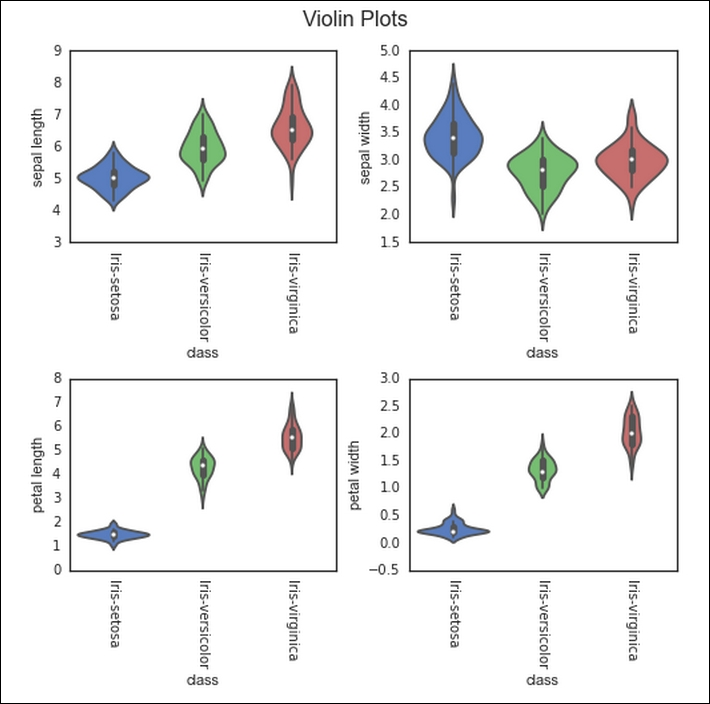Here, we generated a violin plot for each of the four features. A violin plot displays the distribution of the features. For example, we can easily see that the petal length of `iris-setosa` is highly clustered between 1 and 2 cm, while `iris-virginica` is much more dispersed from near 4 to over 7 cm. We can also notice that we have used much of the same code that we used when constructing the `matplotlib` graphs. The main difference is the addition of the `sns.plot()` calls in place of the `ax.plot()` calls previously. We have also added a title above all of the subplots rather than over each individually with the `fig.suptitle()` method. One other notable addition is the iteration over each of the subplots to change the rotation of `xticklabels`. We call `ax.flat()` and then iterate over each subplot axis to set a particular property using `.setp()`. This prevents us from having to individually type out `ax`...`ax` and set the properties as we did in the earlier matplotlib subplot code.

The graphs we've used here are a great start, but there are hundreds of styles of graphs that you can generate using `matplotlib` and `seaborn`. I highly recommend that you dig into the documentation for these two libraries-it will be time well spent.

### Preparation

We've learned a great deal about inspecting the data that we have, but now let's move on to learning how to process and manipulate our data. Here we will learn about the `Series.map()`, `Series.apply()`, `DataFrame.apply()`, `DataFrame.applymap()`, and `DataFrame.groupby()` methods of `pandas`. These are invaluable for working with data and are especially useful in the context of machine learning for feature engineering, a concept that we will discuss in detail in later chapters.

#### Map

The `map` method works on series, so in our case, we will use it to transform a column of our DataFrame, which remember is just a `pandas` Series. Suppose that we decide that the class names are a bit long for our taste and we would like to code them using our special three-letter coding system. We'll use the `map` method with a Python dictionary as the argument to accomplish this. We'll pass in a replacement for each of the unique iris types:

```df['class'] = df['class'].map({'Iris-setosa': 'SET', 'Iris-virginica': 'VIR', 'Iris-versicolor': 'VER'})
df

```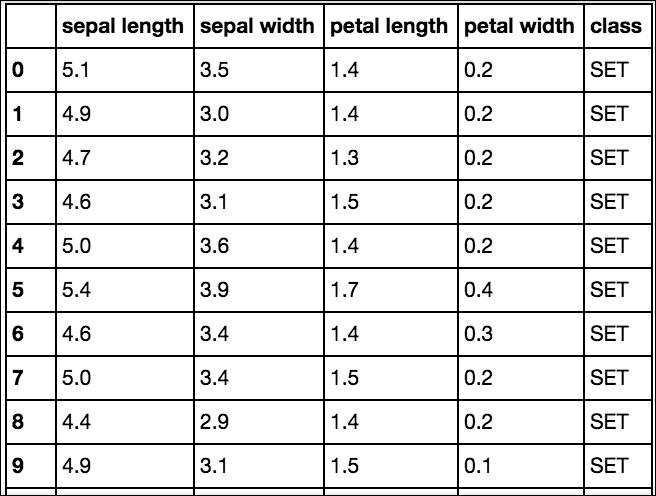Let's look at what we have done here. We ran the `map` method over each of the values of the existing `class` column. As each value was found in the Python dictionary, it was added to the return series. We assigned this return series to the same `class` name, so it replaced our original `class` column. Had we chosen a different name, say `short class`, this column would have been appended to the DataFrame and we would then have the original `class` column plus the new `short class` column.

We could have instead passed another Series or function to the `map` method to perform this transformation on a column, but this is functionality that is also available through the apply method, which we'll take a look at next. The dictionary functionality is unique to the `map` method, and the most common reason to choose `map` over apply for a single column transformation. Let's now take a look at the `apply` method.

#### Apply

The `apply` method allows us to work with both DataFrames and Series. We'll start with an example that would work equally well with `map`, then we'll move on to examples that would work only with `apply`.

Using the `iris` DataFrame, let's make a new column based on the petal width. We previously saw that the mean for the petal width was 1.3. Let's now create a new column in our DataFrame, wide petal, that contains binary values based on the value in the `petal width` column. If the petal width is equal to or wider than the median, we will code it with a 1, and if it is less than the median, we will code it 0. We'll do this using the `apply` method on the `petal width` column:

```df['wide petal'] = df['petal width'].apply(lambda v: 1 if v >= 1.3 else 0)
df
```

The preceding code generates the following output: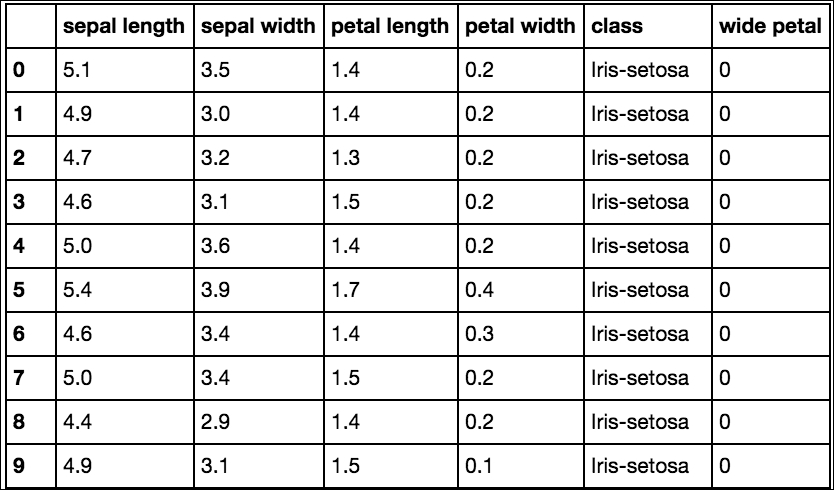A few things happened here; let's walk through them step by step. First, we were able to append a new column to the DataFrame simply using the column selection syntax for a column name that we want to create, in this case, `wide petal`. We set this new column equal to the output of the `apply` method. Here, we ran `apply` on the `petal width` column, which returned the corresponding values in the `wide petal` column. The `apply` method works by running through each value of the `petal width` column. If the value is greater or equal to `1.3`, the function returns `1`; otherwise, it returns `0`. This type of transformation is a fairly common feature-engineering transformation in machine learning, so it is good to be familiar with how to perform it.

Let's now take a look at using apply on a DataFrame rather than a single Series. We'll now create a feature based on `petal area`:

```df['petal area'] = df.apply(lambda r: r['petal length'] * r['petal width'], axis=1)
df
```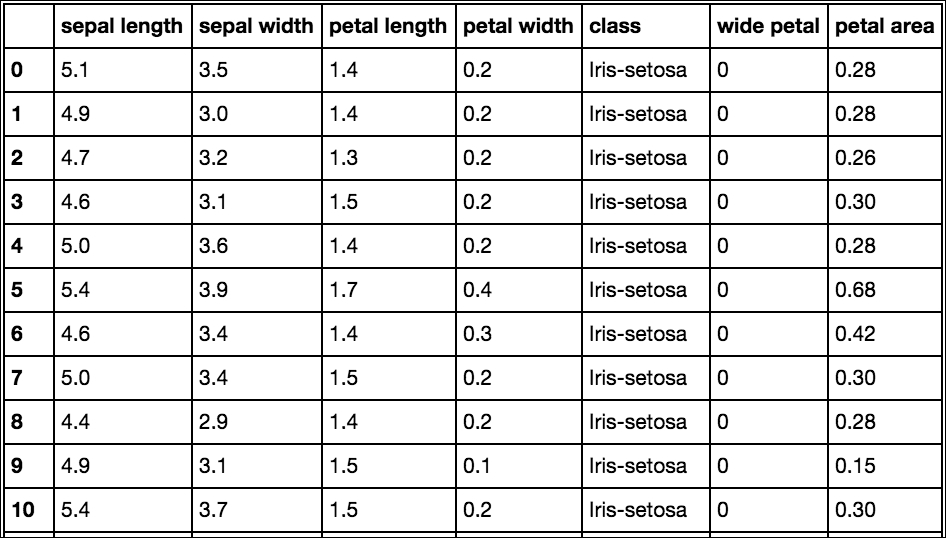Notice that we called `apply`, not on a Series here, but on the entire DataFrame, and because `apply` was called on the entire DataFrame, we passed in `axis=1` in order to tell `pandas` that we want to apply the function row-wise. If we passed in `axis=0`, then the function would operate column-wise. Here, each column is processed sequentially, and we choose to multiply the values from the `petal length` and `petal width` columns. The resultant series then becomes the `petal area` column in our DataFrame. This type of power and flexibility is what makes `pandas` an indispensable tool for data manipulation.

#### Applymap

We've looked at manipulating columns and explained how to work with rows, but suppose that you'd like to perform a function across all data cells in your DataFrame; this is where `applymap` is the right tool. Let's take a look at an example:

```df.applymap(lambda v: np.log(v) if isinstance(v, float) else v)

```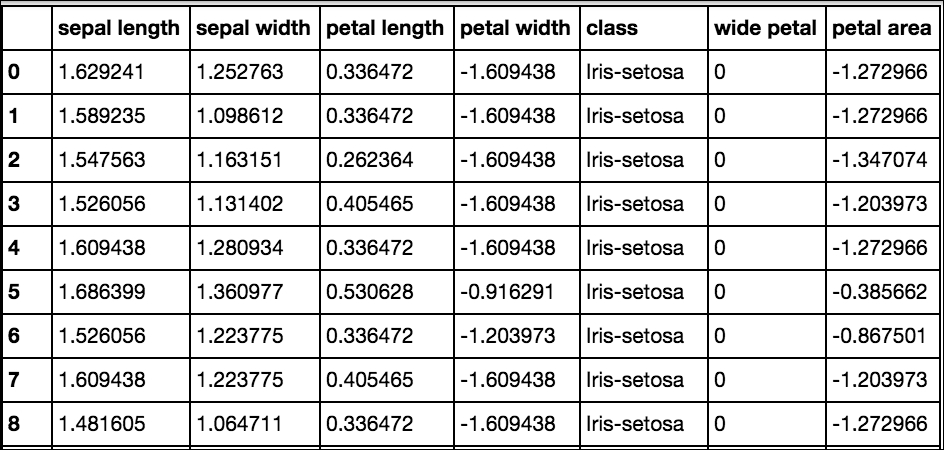Here, we called `applymap` on our DataFrame in order to get the log of every value (`np.log()` utilizes the `numpy` library to return this value) if that value is an instance of the type float. This type checking prevents returning an error or a float for the `class` or `wide petal` columns, which are string and integer values respectively. Common uses for `applymap` are to transform or format each cell based on meeting some conditional criteria.

#### Groupby

Let's now look at an operation that is highly useful but often difficult for new pandas users to get their heads aroundâ€”the DataFrameÂ `.groupby()` method. We'll walk through a number of examples step by step in order to illustrate the most important functionality.

The `groupby` operation does exactly what it saysâ€”it groups data based on some class or classes that you choose. Let's take a look at a simple example using our `iris` dataset. We'll go back and reimport our original `iris` dataset and run our first `groupby` operation:

``` df.groupby('class').mean()
```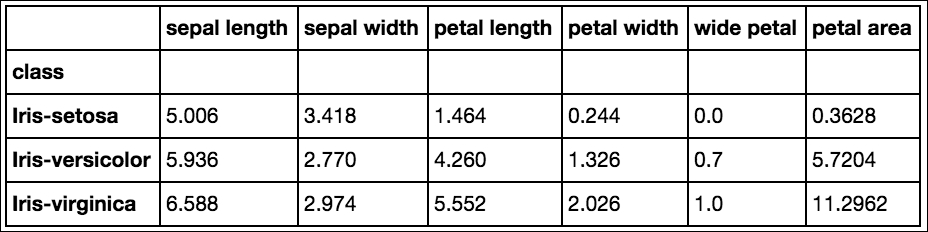Data for each class is partitioned and the mean for each feature is provided. Let's take it a step further now and get full descriptive statistics for each class:

``` df.groupby('class').describe()
```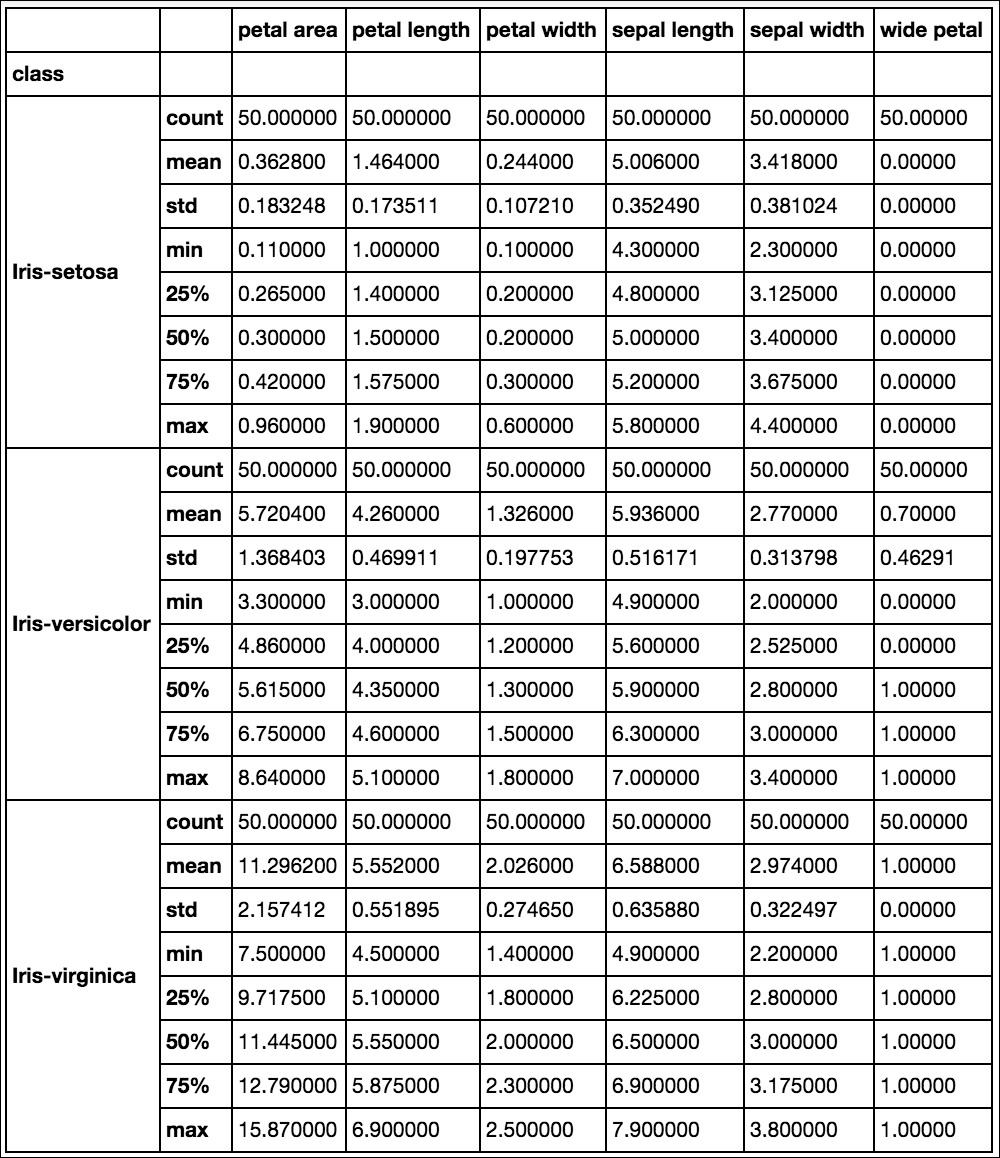Now we can see the full breakdown bucketed by `class`. Let's now look at some other `groupby` operations that we can perform. We saw previously that the petal length and width had some relatively clear boundaries between classes; let's see how we might use `groupby` to see this:

``` df.groupby('petal width')['class'].unique().to_frame()

```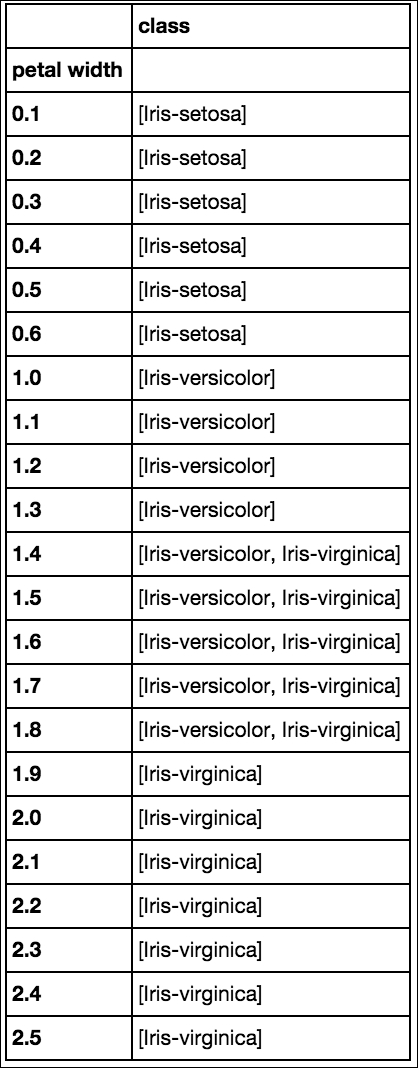In this case, we grouped each unique class by the petal width that they were associated with. This is a manageable number of measurements to group by, but if it were to become much larger, we would likely need to partition the measurements into brackets. As we saw previously, this can be accomplished with the `apply` method.

Let's now take a look at a custom aggregation function:

```df.groupby('class')['petal width']\
.agg({'delta': lambda x: x.max() - x.min(), 'max': np.max, 'min': np.min})

```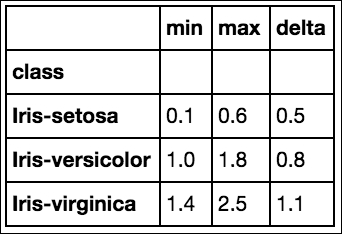In this code, we grouped the petal width by class using the functions: `np.max` and `np.min`, and a `lambda` function that returns the maximum petal width minus the minimum petal width. (The two `np` functions are from the `numpy` library.) These were passed to the `.agg()` method in the form of a dictionary in order to return a DataFrame with the keys as column names. A single function can be run or the functions can be passed as a list, but the column names are less informative.

### Note

We've only just touched on the functionality of the `groupby` method; there is a lot more to learn, so I encourage you to read the documentation at http://pandas.pydata.org/pandas-docs/stable/.

We now have a solid base-level understanding of how to manipulate and prepare data in preparation for our next step, which is modeling. We will now move on to discuss the primary libraries in the Python machine learning ecosystem.

### Modeling and evaluation

Python has an excellent selection of well-documented libraries for statistical modeling and machine learning. We'll touch on just a few of the most popular libraries below.

#### Statsmodels

The first library that we'll cover is the `statsmodels` library (http://statsmodels.sourceforge.net/). Statsmodels is a Python package that was developed to explore data, estimate models, and run statistical tests. Let's use it here to build a simple linear regression model of the relationship between the sepal length and sepal width for the `setosa` class.

First, let's visually inspect the relationship with a scatterplot:

```fig, ax = plt.subplots(figsize=(7,7))
ax.scatter(df['sepal width'][:50], df['sepal length'][:50])
ax.set_ylabel('Sepal Length')
ax.set_xlabel('Sepal Width')
ax.set_title('Setosa Sepal Width vs. Sepal Length', fontsize=14,
y=1.02)
```

The preceding code generates the following output: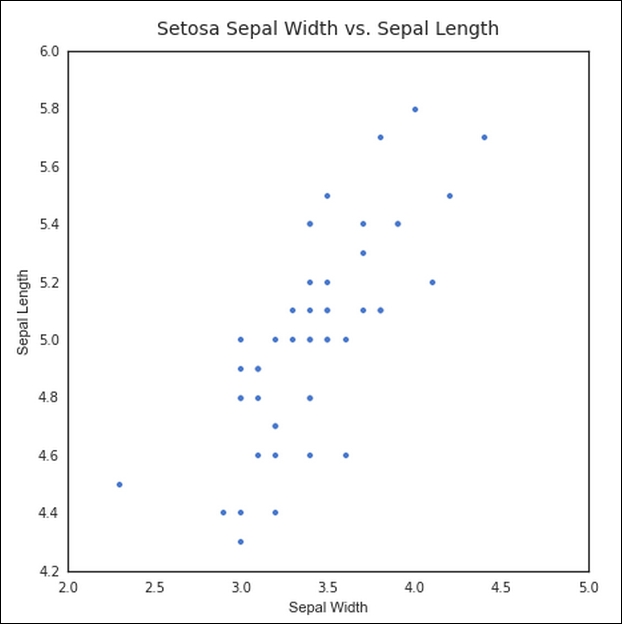We can see that there appears to be a positive linear relationship, that is, as the sepal width increases, sepal length does as well. We next run a linear regression model on the data using `statsmodels` to estimate the strength of this relationship:

```import statsmodels.api as sm

y = df['sepal length'][:50]
x = df['sepal width'][:50]

results = sm.OLS(y, X).fit()
print(results.summary())

```

The preceding code generates the following output: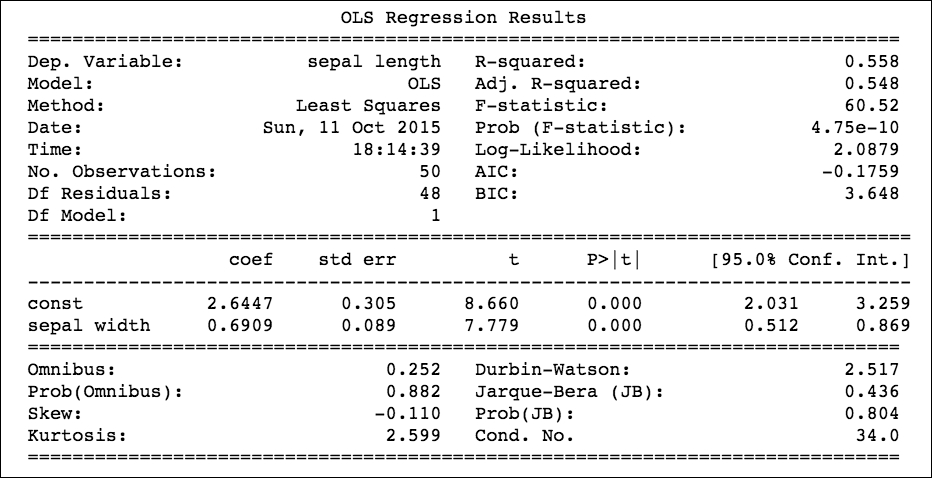The preceding screenshot shows the results of our simple regression model. As this is a linear regression, the model takes the format of Y = Î’0+Î’1X, where B0 is the intercept and B1 is the regression coefficient. Here, the formula would be Sepal Length = 2.6447 + 0.6909 * Sepal Width. We can also see that R2 for the model is a respectable `0.558`, and the p-value (`Prob`) is highly significantâ€”at least for this class.

Let's now use the results object to plot our regression line:

```fig, ax = plt.subplots(figsize=(7,7))
ax.plot(x, results.fittedvalues, label='regression line')
ax.scatter(x, y, label='data point', color='r')
ax.set_ylabel('Sepal Length')
ax.set_xlabel('Sepal Width')
ax.set_title('Setosa Sepal Width vs. Sepal Length', fontsize=14,
y=1.02)
ax.legend(loc=2)

```

The preceding code generates the following output: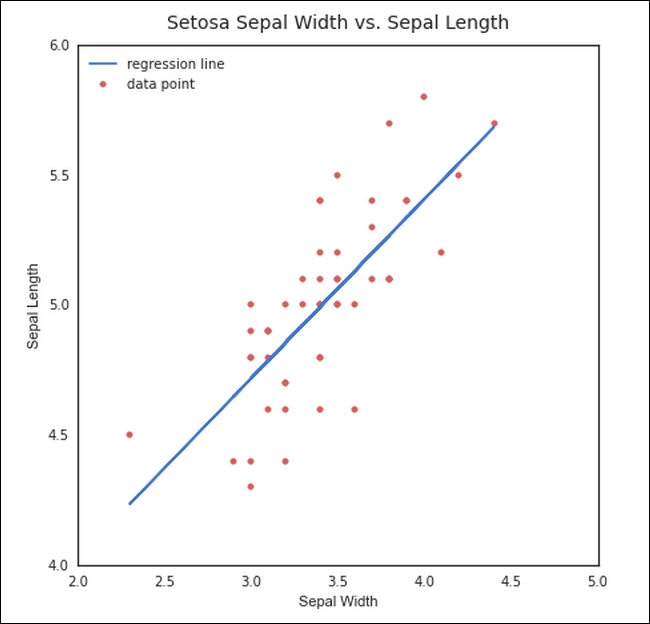By plotting `results.fittedvalues`, we can get the resulting regression line from our model.

There are a number of other statistical functions and tests in the `statsmodels` package, and I invite you to explore them. It is an exceptionally useful package for standard statistical modeling in Python. Let's now move on to the king of Python machine learning packages, `scikit-learn`.

#### Scikit-learn

Scikit-learn is an amazing Python library with unrivaled documentation designed to provide a consistent API to dozens of algorithms. It is built onâ€”and is itselfâ€”a core component of the Python scientific stack, namely, NumPy, SciPy, pandas, and matplotlib. Here are some of the areas that scikit-learn covers: classification, regression, clustering, dimensionality reduction, model selection, and preprocessing.

We'll look at a few examples. First, we will build a classifier using our `iris` data, and then we'll look at how we can evaluate our model using the tools of scikit-learn.

The first step to building a machine learning model in scikit-learn is understanding how the data must be structured. The independent variables should be a numeric n x m matrix, `X`;Â a dependent variable, `y`;Â and an n x 1 vector. The y vector may be either numeric continuous or categorical or string categorical. These are then passed into the `.fit()` method on the chosen classifier. This is the great benefit of using scikit-learn; each classifier utilizes the same methods to the extent that's possible. This makes swapping them in and out a breeze. Let's see this in action in our first example:

```from sklearn.ensemble import RandomForestClassifier
from sklearn.cross_validation import train_test_split

clf = RandomForestClassifier(max_depth=5, n_estimators=10)

X = df.ix[:,:4]
y = df.ix[:,4]

X_train, X_test, y_train, y_test = train_test_split(X, y,
test_size=.3)

clf.fit(X_train,y_train)

y_pred = clf.predict(X_test)

rf = pd.DataFrame(list(zip(y_pred, y_test)), columns=['predicted',
'actual'])
rf['correct'] = rf.apply(lambda r: 1 if r['predicted'] ==
r['actual'] else 0, axis=1)

rf

```

The preceding code generates the following output: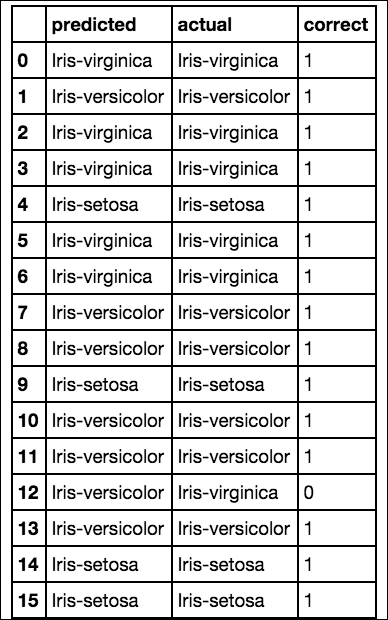Now, let's take a look at the following line of code:

```rf['correct'].sum()/rf['correct'].count()
```

This will generate the following output: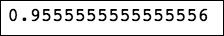In the preceding few lines of code, we built, trained, and tested a classifier that has a 95% accuracy level on our Iris dataset. Let's unpack each of the steps. In the first two lines of code, we made a couple of imports; the first two are from scikit-learn, which, thankfully, is shortened to `sklearn` in import statements. The first import is a random forest classifier, and the second is a module to split our data into training and testing cohorts. This data partitioning is critical in building machine learning applications for a number of reasons. We'll get into this in later chapters, but suffice it to say for now that it is a must. This `train_test_split` module also shuffles our data, which again is important as the order can contain information that would bias your actual predictions

### Note

In this book we'll be using the latest Python version, as of the time of writing, which is version 3.5. If you are on Python version 2.X, you will need to add an additional import statement for integer division to work as it does in Python 3.X. Without this line, your accuracy will be reported as 0 rather than 95%. That line is as follows:

from __future__ import division

The first curious-looking line after the imports instantiates our classifier, in this case, a random forest classifier. We select a forest that uses 10 decision trees, and each tree is allowed a maximum split depth of five. This is put in place to avoid overfitting, something that we will discuss in depth in later chapters.

The next two lines create our `X` matrix and `y`Â vector. Our original `iris` DataFrame contained four features: the petal width and length and the sepal width and length. These features are selected and become our independent feature matrix, `X`. The last column, the `iris` class names, then become our dependent `y` vector.

These are then passed into the `train_test_split` method, which shuffles and partitions our data into four subsets, `X_train`, `X_test`, `y_train`, and `y_test`. The `test_size` parameter is set to `.3`, which means that 30% of our dataset will be allocated to the `X_test` and `y_test` partitions, while the rest will be allocated to the training partitions, `X_train` and `y_train`.

Next, our model is fit using the training data. Having trained the model, we then call the predict method on our classifier using our test data. Remember that the test data is data that the classifier has not seen. The return of this prediction is a list of prediction labels. We then create a DataFrame of the actual labels versus the predicted labels. We finally sum the correct predictions and divide by the total number of instances, which we can see gave us a very accurate prediction. Let's now see which features gave us the most discriminative or predictive power:

```f_importances = clf.feature_importances_f_names = df.columns[:4]
f_std = np.std([tree.feature_importances_ for tree in
clf.estimators_], axis=0)

zz = zip(f_importances, f_names, f_std)
zzs = sorted(zz, key=lambda x: x, reverse=True)

imps = [x for x in zzs]
labels = [x for x in zzs]
errs = [x for x in zzs]

plt.bar(range(len(f_importances)), imps, color="r", yerr=errs,
align="center")
plt.xticks(range(len(f_importances)), labels);

```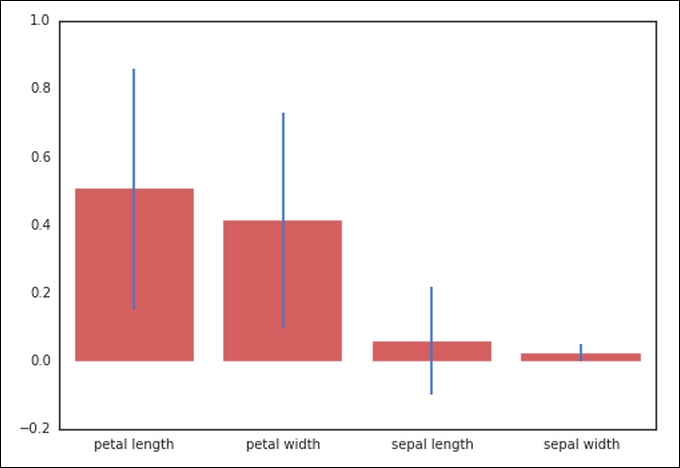As we expected, based upon our earlier visual analysis, the petal length and width have more discriminative power when differentiating between the `iris` classes. Where exactly did these numbers come from though? The random forest has a method called `.feature_importances_` that returns the relative power of the feature to split at the leaves. If a feature is able to consistently and cleanly split a group into distinct classes, it will have a high feature importance. This number will always sum to one. As you will notice here, we have included the standard deviation, which helps to illustrate how consistent each feature is. This is generated by taking the feature importance for each of the features for each ten trees and calculating the standard deviation.

Let's now take a look at one more example using scikit-learn. We will now switch our classifier and use a support vector machine (SVM):

```from sklearn.multiclass import OneVsRestClassifier
from sklearn.svm import SVC
from sklearn.cross_validation import train_test_split

clf = OneVsRestClassifier(SVC(kernel='linear'))

X = df.ix[:,:4]
y = np.array(df.ix[:,4]).astype(str)

X_train, X_test, y_train, y_test = train_test_split(X, y,
test_size=.3)

clf.fit(X_train,y_train)

y_pred = clf.predict(X_test)

rf = pd.DataFrame(list(zip(y_pred, y_test)), columns=['predicted',
'actual'])
rf['correct'] = rf.apply(lambda r: 1 if r['predicted'] ==
r['actual'] else 0, axis=1)

rf

```

The preceding code generates the following output: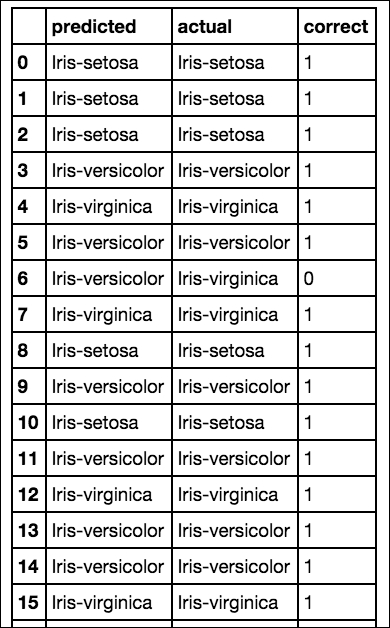Now, let's execute the following line of code:

```rf['correct'].sum()/rf['correct'].count()
```

The preceding code generates the following output: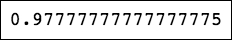Here, we have swapped in a support vector machine without changing virtually any of our code. The only changes were the ones related to the importing of the SVM instead of the random forest, and the line that instantiates the classifier. (One small change to the format of the `y` labels was required, as the SVM wasn't able to interpret them as NumPy strings like the random forest classifier was).

This is just a fraction of the capability of scikit-learn, but it should serve to illustrate the functionality and power of this magnificent tool for machine learning applications. There are a number of additional machine learning libraries that we won't have a chance to discuss here but will explore in later chapters, but I strongly suggest that if this is your first time utilizing a machine learning library and you want a strong general purpose tool, scikit-learn is your go-to choice.

### Deployment

There are a number of options available when putting a machine learning model into production. It depends substantially on the nature of the application. Deployment could include anything from a cron job run on your local machine to a full-scale implementation deployed on an Amazon EC2 instance.

We won't go into detail about specific implementations here, but we will have a chance to delve into different deployment examples throughout the book.

## Setting up your machine learning environment

We've covered a number of libraries throughout this chapter that can be installed individually with pip,Â Python's package manager. I would strongly urge you, however, to go with a prepacked solution such as Continuum's Anaconda Python distribution. This is a a single executable that contains nearly all the packages and dependencies needed. And because the distribution is targeted to Python scientific stack users, it is essentially a one-and-done solution.

Anaconda also includes a package manager that makes updating packages a simple task. Simply type `conda update <package_name>`, and the library will be updated to the most recent stable release.

## Summary

In this chapter, we introduced the data science/machine learning workflow. We saw how to take our data step by step through each stage of the pipeline, going from acquisition all the way through to deployment. We also covered key features of each of the most important libraries in the Python scientific stack.

We will now take this knowledge and these lessons and begin to apply them to create unique and useful machine learning applications. In the next chapter, we'll see how we can apply regression modeling to find a cheap apartment. Let's get started!